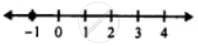# For the Following Inequation, Represent the Solution on a Number Line : 4(3x + 1) > 2(4x - 1), X is a Negative Integer - Mathematics

Sum

For the following inequation, represent the solution on a number line :

4(3x + 1) > 2(4x - 1), x is a negative integer

#### Solution

4(3x + 1) > 2(4x - 1), x is a negative integer

⇒ 12x + 4 > 8x - 2

⇒ 12x - 8x > -2 - 4

⇒ 4x > -6

⇒ x > (-6)/4

⇒ x > -1.5

∴ x = {-1}Concept: Operation on of Whole Number on Number Line
Is there an error in this question or solution?

#### APPEARS IN

Selina Concise Mathematics Class 8 ICSE
Chapter 15 Linear Inequations
Exercise 15 (B) | Q 13.4 | Page 175